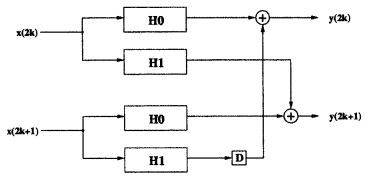## How to parallelize polyphase FIR resampling filtersStarted by 3 years ago7 replieslatest reply 3 years ago591 views

I am struggling to find a good reference for implementing parallel rational-ratio FIR resampling filters. By "parallel", I mean a high-throughput hardware filter with P parallel input samples and Q parallel output samples:Note that P and Q are not necessarily coprime, so we have divided out any common factors to get coprime M and L as required for polyphase decomposition. In other words, (output sample rate)/(input sample rate) = Q/PL/M.

In the "integer-ratio" case (where either P = 1 or Q = 1), I can see how standard polyphase representations map very naturally into parallel hardware. For example, we can just discard the circuitry that interleaves the output samples of a 1:L polyphase interpolator, and we are left with a parallel implementation (similar applies for M:1 decimator):However, in the general M:L case, the procedure for parallelization is not so clear. In the book "Multirate Digital Signal Processing" by Fliege, he provides a superb pictorial derivation of the M:L polyphase decomposition, but with a focus on efficiency that doesn't map directly into the parallel P:Q structure drawn above (even if we assume PM and QL are coprime):So, I derived a few special cases by hand, identified a pattern, and confirmed (in Matlab) that the pattern is valid for every combination of P and Q that I had the patience to test. However, it bothers me that this is not rigorous. It also bothers me that even the simple special cases were diabolically complicated to derive (via the method I used) and yet the final structure is extremely simple.

Therefore, my question is whether anyone can point me to a proper derivation. (Preferably, an excellent pictorial one in the style of Fliege!) The closest I have found are:

• A "DSP Tips&Tricks" article by Douglas W. Barker in the July 2008 edition of IEEE Signal Processing Magazine, entitled "Efficient Resampling Implementations". This describes a simple procedure that is possibly equivalent to what I have done, but with no derivation or explicit description of the general case.
• In Section 9.2.1 of "VLSI Digital Signal Processing Systems" by Keshab K. Parhi, the "non-resampling" parallel FIR filter (P = Q) is derived. However, there aren't many pictures and I find the equations rather difficult to digest.

Many thanks in advance for any suggestions!

[ - ]Are you trying to optimize for a fixed resample rate or are you trying to find a good architecture for a flexible multi-rate resampling filter?

The multi-rate problem is pretty well studied and there are some good practical architectures that will do pretty much any resampling rate.   In digital comm this is done to recover symbols that will have random phase offsets as well as frequency drift that must be tracked.

[ - ]@ Fixed resample rate. The resample rate can even be 1. For example, the most popular structure in the literature seems to be the "two-parallel FIR filter" (P = Q = 2):In this case, there is no rate change (M = L = 1). It's just a parallelized FIR filter.

[ - ]I will describe my thoughts (in words rather than picture or formula):

your single rate parallelized case of two subfilters is the starting point.

1) you can nest that structure as many times as required.

2) For rational structure of I/D (Interpolation/Decimation) I can insert (I) zeros (not physically needed, as all subfilters on such paths will give zero outputs). At final outputs I will discard every D-1 samples taking into account the zero filter cases.

Thus your final rate = I/D

[ - ]@ I don't think I follow your explanation. Could you give a simple example? For example, if I want 6 parallel inputs and 8 parallel outputs (interpolation by 4, decimation by 3), how would you construct that filter? And how does it then generalize for any P and Q?

[ - ]The principle is same as having single rate filter when used to interpolate/decimate directly (without polyphase approach) i.e. insert I-1 zeros then filter then decimate D-1 from output.

so in case of parallel paths implement zero insertion, your filter then decimate in the same way.

assume your original samples are s0,s1,s2...etc

A)zero insertion:

insert 3 zeros after each sample (s0,0,0,s1,0,0,0,s2,0,0...etc)

B) split original stream based on even/odd samples(two paths):

even stream: s0,0,0,0,0,...

odd stream:  0,s1,0,s2,0,...

if you wish split each of even/odd streams further into even/odd to get four paths or split further into more paths.

C) apply filtering as per your 2 x 2 subfilters diagram on every pair of input streams. filters with zero inputs need not be computed but a zero output is implied. Take care of Delay factor in the output adders.

D) discard D-1 samples at output starting from first sample (normally phase 1 of output), each sample can now go into one of 8 parallel streams

[ - ]@ I understand the principle of rational-ratio resampling, but the challenge here lies in determining (in closed form) where the redundancy will be. Or, equivalently, where the required (non-redundant) operations will be. This is exactly the same challenge as in conventional polyphase decomposition, but with the extra constraint of parallel interfaces.

I found a couple of tricks that allowed me to finish the general proof that confirms that the structure I found is correct. However, I would still really like to find a textbook or paper that covers this. I would have thought that hundreds of people would have implemented these kinds of filters, so it's frustrating that I can't find anything.

[ - ]